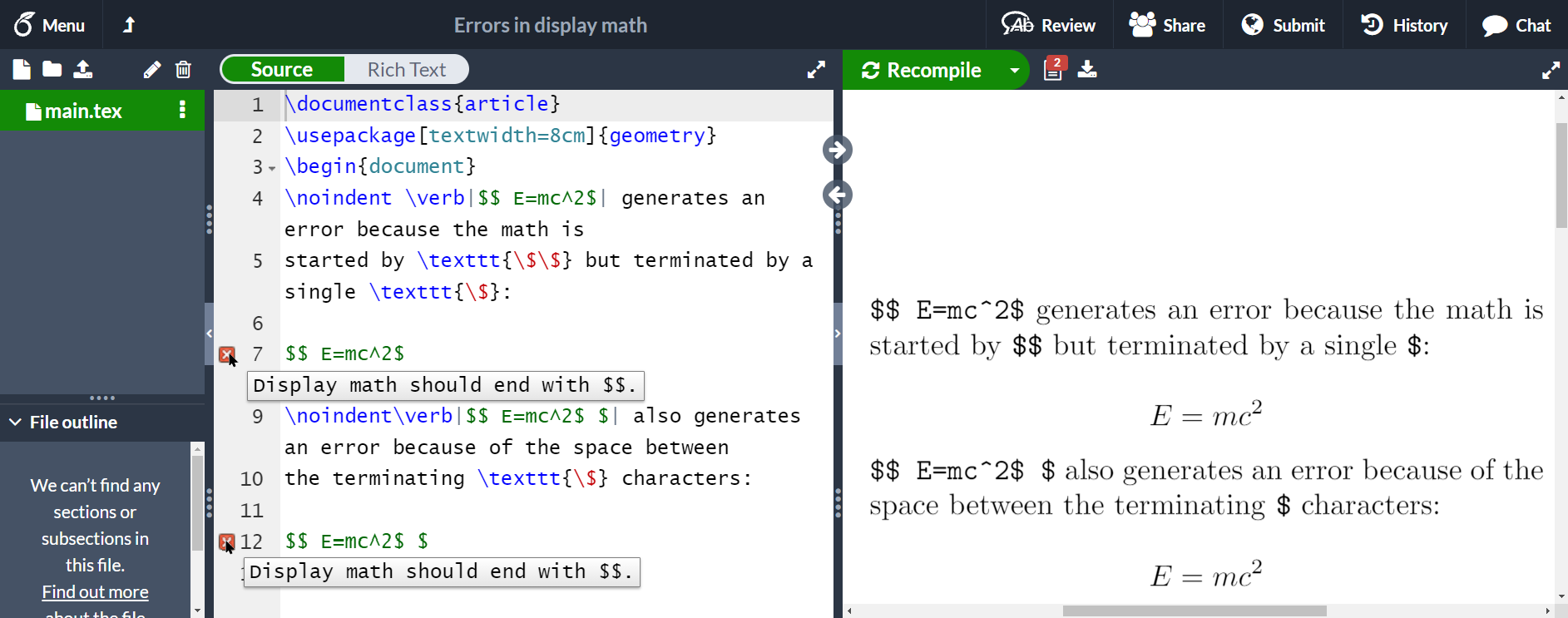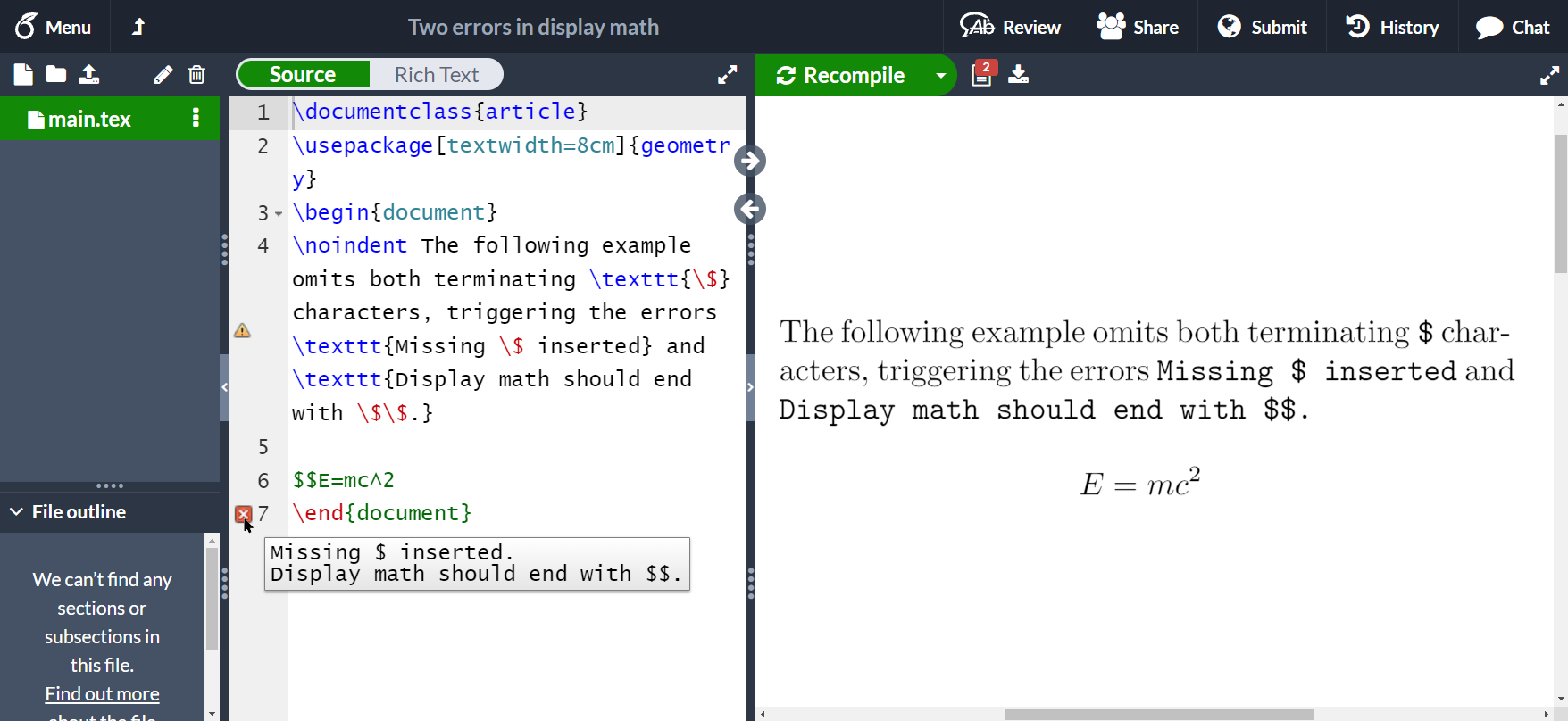# Introduction

TeX engines have two ways of typesetting mathematics:

• inline math mode where the mathematical content is contained within a paragraph, and
• display math mode where mathematical material is displayed separately, with additional space above or below it.

Traditionally, in the early days of TeX, mathematics intended to be typeset inline, typically within a paragraph, was surrounded by single $ characters: $ inline math content...$ and mathematics destined for display was surrounded by double $ characters: $$display math content...$$.

# Cause of the error Display math should end with $$ The error message Display math should end with$$ is generated by TeX engines when they try to finish typesetting some display math material but are unable to cleanly exit from display math mode due to incorrect TeX markup: as the error message indicates, the material to be typeset as display math has not been terminated with a second $$ pair. ## Examples: single error This error is demonstrated in the following examples: \documentclass{article} \usepackage[textwidth=8cm]{geometry} \begin{document} \noindent \verb|$$ E=mc^2$| generates an error because the math is started by \texttt{\$\$} but terminated by a single \texttt{\$}:

$$E=mc^2 \noindent\verb|$$ E=mc^2| also generates an error because of the space between
the terminating \texttt{\$} characters: $$E=mc^2 \end{document}  This example produces the following output (image edited to highlight both errors):## Example: two errors Note: In some circumstances you may also see the related error Missing inserted, as the following example demonstrates by writing $$E=mc^2, which omits both terminating $ characters:

\documentclass{article}
\usepackage[textwidth=8cm]{geometry}
\begin{document}
\noindent The following example omits both terminating \texttt{\$} characters, triggering the errors \texttt{Missing \$ inserted} and \texttt{Display math should end with \$\$.}

$$E=mc^2 \end{document}  This example produces the following output:# Solution For the errors demonstrated above, the fix is straightforward—make sure you add the closing $$ at the end of your display math:

\documentclass{article}
\begin{document}
\noindent The solution is to ensure correct termination of the
display math by writing \verb|$$E=mc^2$$|:

$$E=mc^2$$
\end{document}


# Avoid using $ characters to typeset mathematics Nowadays, standard (accepted) best practice is to avoid using explicit $ characters to typeset mathematics and use LaTeX delimiters instead, particularly for display math:

• for display math: write $display math content$ instead of $$display math content...$$
• for inline math: write $$inline math content$$ instead of $inline math content...$

In reality, the LaTeX delimiters $$, $$, $ and $ are single-character macros which provide a sort of “insulating wrapper” around single and double $ characters. The LaTeX definitions of those delimiters (macros) do actually contain $ characters but with additional code that runs some tests/checks. They also generate LaTeX’s error message Bad math environment delimiter. Using these delimiters (macros) has additional advantages because they can be redefined, perhaps temporarily, to achieve special effects.